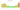# MKD

## Macedonian Denar

#### how has the value of the currency changed in the last year?## USD 1 = MKD 58.339

How much is10 US dollars worth inMacedonian denari?
At the current exchange rate, 10 US dollars is worth 583.39 Macedonian denari
How much is50 US dollars worth inMacedonian denari?
At the current exchange rate, 50 US dollars is worth 2,916.96 Macedonian denari
How much is100 US dollars worth inMacedonian denari?
At the current exchange rate, 100 US dollars is worth 5,833.93 Macedonian denari
How much is500 US dollars worth inMacedonian denari?
At the current exchange rate, 500 US dollars is worth 29,169.63 Macedonian denari
How much is2,000 US dollars worth inMacedonian denari?
At the current exchange rate, 2,000 US dollars is worth 116,678.53 Macedonian denari
How much is10 US dollars worth inMacedonian denari?
At the current exchange rate, 10 US dollars is worth 583.39 Macedonian denari
How much is50 US dollars worth inMacedonian denari?
At the current exchange rate, 50 US dollars is worth 2,916.96 Macedonian denari
How much is100 US dollars worth inMacedonian denari?
At the current exchange rate, 100 US dollars is worth 5,833.93 Macedonian denari
How much is500 US dollars worth inMacedonian denari?
At the current exchange rate, 500 US dollars is worth 29,169.63 Macedonian denari
How much is2,000 US dollars worth inMacedonian denari?
At the current exchange rate, 2,000 US dollars is worth 116,678.53 Macedonian denari